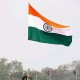SearchSearch

# Top 25 Latest Elementary Mathematics MCQs for NDA 2 2017 Exam

As per the latest syllabus of NDA 2017 entry, we are sharing Top 25 Latest Elementary Mathematics MCQs for NDA 2 2017 Exam . Appearing candidates must see.## Top 25 Latest Elementary Mathematics MCQs for NDA 2 2017 Exam1.  The LCM of two numbers is 280 and their ratio is 7:8. The two numbers are a. 70, 80 b. 35, 40 c. 42, 48 d. 28,32
2.  If  n  is a positive integer, which one of the following numbers must have a remainder of 3 when divided by any of the numbers 4, 5 and 6? a. 12n+3 b. 24n+3 c. 90n+2 d. 120n+3
3.  What is the remainder left after dividing 1!+2!+3!…..+100! by 77? a. 0 b. 5 c. 14 d. 21
4.  The LCM of two number is 4800 and their HCF is 160. If one number is 480, then the second number is: a. 1200 b. 1500 c. 1600 d. 1800
5.  How many natural numbers below 660 are divisible by 5 and 11 but not by 3? a. 8 b. 9 c. 10 d. 11
6.  Four digits of the number 29138576 are omitted so that the result is as large as possible. The largest omitted digit is: a. 5 b. 6 c. 7 d. 8
7.  A two-digit number is such that the product of the digits is 8. When 18 is added to the number, then the digits are reversed. The number is: a. 18 b. 24 c. 42 d. 81
8.  – 496157 x 9999 = y. What is y? a. – 4691100813 b. – 4691100843 c. -4691100823 d. -4691100833
9.  The cube root of .000216 is: a. .6 b. .06 c. 77 d. 87
10.  The square root of (7 + 3√5) (7 – 3√5) is a. √5 b. 2 c. 4 d. .3√5
11.  A group of students decided to collect as many paise from each member of group as is the number of members. If the total collection amounts to Rs. 59.29, the number of the member is the group is: a. 57 b. 67 c. 77 d. 87
12.  If one third of one fourth of number is 15, then three tenth of number is a. 34 b. 44 c. 54 d. 64
13.  An athlete runs 200 meters in 24 seconds. His speed is ? a. 10 km/hr b. 17 k/hr c. 27 km/hr d. 30 km/hr
14.  The distance between two cities A and B is 330 Km. A train starts from A at 8 a.m. and travel towards B at 60 km/hr. Another train starts from B at 9 a.m and travels towards A at 75 Km/hr. At what time do they meet? a. 10 am b. 11 am c. 12 pm d. 1 pm
15.  A thief is noticed by a policeman from a distance of 200 m. The thief starts running and the policeman chases him. The thief and the policeman run at the rate of 10 km and 11 km per hour respectively. What is the distance between them after 6 minutes ? a. 50 meter b. 100 meter c. 110 meter d. None of above
16.  In a flight of 600 km, an aircraft was slowed down due to bad weather. Its average speed for the trip was reduced by 200 km/hr and the time of flight increased by 30 minutes. The duration of the flight is: a. 1 hour b. 2 hours c. 3 hours d. 4 hours
17.  A man on tour travels first 160 km at 64 km/hr and the next 160 km at 80 km/hr. The average speed for the first 320 km of the tour is: a. 35.55 km/hr b. 36 km/hr c. 71.11 km/hr d. 71 km/hr
18.  It takes eight hours for a 600 km journey, if 120 km is done by train and the rest by car. It takes 20 minutes more, if 200 km is done by train and the rest by car. The ratio of the speed of the train to that of the cars is: a. 2 : 3 b. 3 : 2 c. 3 : 4 d. 4 : 3
19.  A farmer travelled a distance of 61 km in 9 hours. He travelled partly on foot @ 4 km/hr and partly on bicycle @ 9 km/hr. The distance travelled on foot is: a. 14 km b. 15 km c. 16 km d. 17 km
20.  Two persons starting from the same place walk at a rate of 5kmph and 5.5kmph respectively. What time will they take to be 8.5km apart, if they walk in the same direction? a. 17 hrs b. 22 hrs c. 25 hrs d. 12 hrs
21.  Rahul and Sham together can complete a task in 35 days, but Rahul alone can complete same work in 60 days. Calculate in how many days Sham can complete this work ? a. 82 days b. 84 days c. 68 days d. 76 days
22.  Twenty-four men can complete a work in sixteen days. Thirty-two women can complete the same work in twenty-four days. Sixteen men and sixteen women started working and worked for twelve days. How many more men are to be added to complete the remaining work in 2 days? a. 18 men b. 24 men c. 50 men d. 36 men
23.  A is 30% more efficient than B. How much time will they, working together, take to complete a job which A alone could have done in 23 days? a. 20(3/17) days b. 13 days c. 11 days d. None of these
24.  45% of 600 is equal to: a. 300 b. 270 c. 350 d. 250
25.  The price of a book increases by 10% in June but in July it decreases by 10%. What is its price at the end of July if its original price was 200? a. 190 b. 200 c. 210 d. 198
• Comment (1)
•dheeraj patidar says:

psl sir answers should be there……